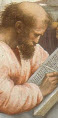# Pythagoras' Theorem in 3D

## In 2D

First, let us have a quick refresher in two dimensions:Pythagoras

When a triangle has a right angle (90°) ...

... and squares are made on each of the three sides, ...

images/pyth1.js

... then the biggest square has the exact same area as the other two squares put together!It is called "Pythagoras' Theorem" and can be written in one short equation:

a2 + b2 = c2Note:

• c is the longest side of the triangle
• a and b are the other two sides

And when we want to know the distance "c" we take the square root:

c2 = a2 + b2

c = √(a2 + b2)

You can read more about it at Pythagoras' Theorem, but here we see how it can be extended into 3 Dimensions.

## In 3D

Let's say we want the distance from the bottom-most left front corner to the top-most right back corner of this cuboid:First let's just do the triangle on the bottom.

Pythagoras tells us that c = √(x2 + y2)Now we make another triangle with its base along the "√(x2 + y2)" side of the previous triangle, and going up to the far corner:We can use Pythagoras again, but this time the two sides are √(x2 + y2) and z, and we get this formula:And the final result is:So it is all part of a pattern that extends onwards:

Dimensions Pythagoras Distance "c"
1 c2 = x2 √(x2) = x
2 c2 = x2 + y2 √(x2 + y2)
3 c2 = x2 + y2 + z2 √(x2 + y2 + z2)
... ... ...
n c2 = a12 + a22 + ... + an2 √(a12 + a22 + ... + an2)

So next time you need an n-dimensional distance you will know how to calculate it!